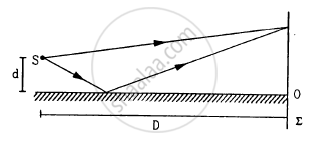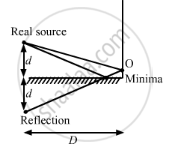Department of Pre-University Education, KarnatakaPUC Karnataka Science Class 12
Advertisement Remove all ads

# A Narrow Slit S Transmitting Light of Wavelength λ is Placed a Distance D Above a Large Plane Mirror, as Shown in the Following Figure. - Physics

Sum

A narrow slit S transmitting light of wavelength λ is placed a distance d above a large plane mirror, as shown in the following figure. The light coming directly from the slit and that coming after the reflection interfere at a screen ∑ placed at a distance D from the slit. (a) What will be the intensity at a point just above the mirror, i.e. just above O? (b) At what distance from O does the first maximum occur?Advertisement Remove all ads

#### Solution

(a) The phase of a light wave reflecting from a surface differs by $\pi$ from the light directly coming from the source.Thus, the wave fronts reaching just above the mirror directly from the source and after reflecting from the mirror have a phase difference of $\pi,$ which is the condition of distractive interference. So, the intensity at a point just above the mirror is zero.

(b) Here, separation between two slits is 2d.

Wavelength of the light is lambda.

Distance of the screen from the slit is D.

Consider that the bright fringe is formed at position y. Then,

path difference, $∆ x = \frac{y \times 2d}{D} = n\lambda.$

After reflection from the mirror, path difference between two waves is $\frac{\lambda}{2}.$

$\Rightarrow \frac{y \times 2d}{D} = \frac{\lambda}{2} + n\lambda$

For first order, put n = 0

$\Rightarrow y = \frac{\lambda D}{4d}$

Concept: Interference
Is there an error in this question or solution?
Advertisement Remove all ads

#### APPEARS IN

HC Verma Class 11, 12 Concepts of Physics 1
Chapter 17 Light Waves
Q 21 | Page 381
Advertisement Remove all ads

#### Video TutorialsVIEW ALL 

Advertisement Remove all ads
Share
Notifications

View all notifications

Forgot password?